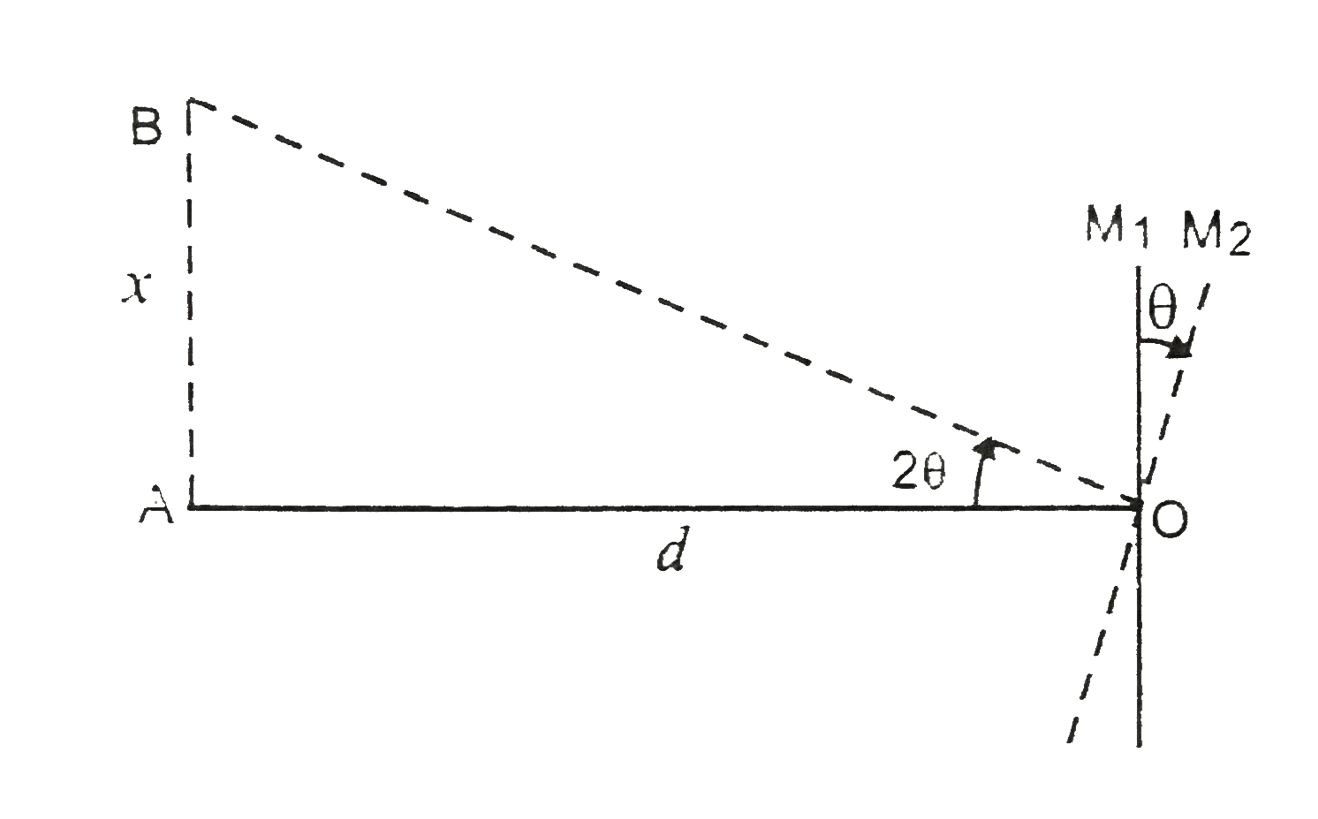# Light incident normally on a plane mirror attached to a galvanometer coil retraces backwards a shown in Fig. 6(a). 14. A current in the coil produces

29 views
in Physics
closed
Light incident normally on a plane mirror attached to a galvanometer coil retraces backwards a shown in Fig. 6(a). 14. A current in the coil produces a deflection of 3.5^(@) in the mirror. What is the displacement of the reflected spot of light on a screen placed 1.5 m away ?

by (86.1k points)
selected

In Fig., when mirror is turned from position M_1 to position M_2 through /_theta = 3.5^@, the reflected ray turns through
/_2 theta = 7^@ = /_AOB
OA = d = 1.5 m
Displacement, AB = x = ?
In Delta AOB tan 2 theta = (x)/(d)
x = d tan 2 theta = 1.5 tan 7^@ = 1.5 xx 0.1228
=0.18 metre..